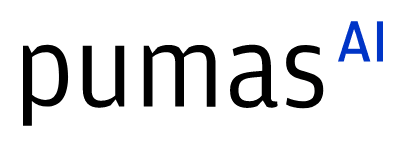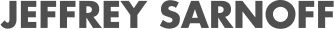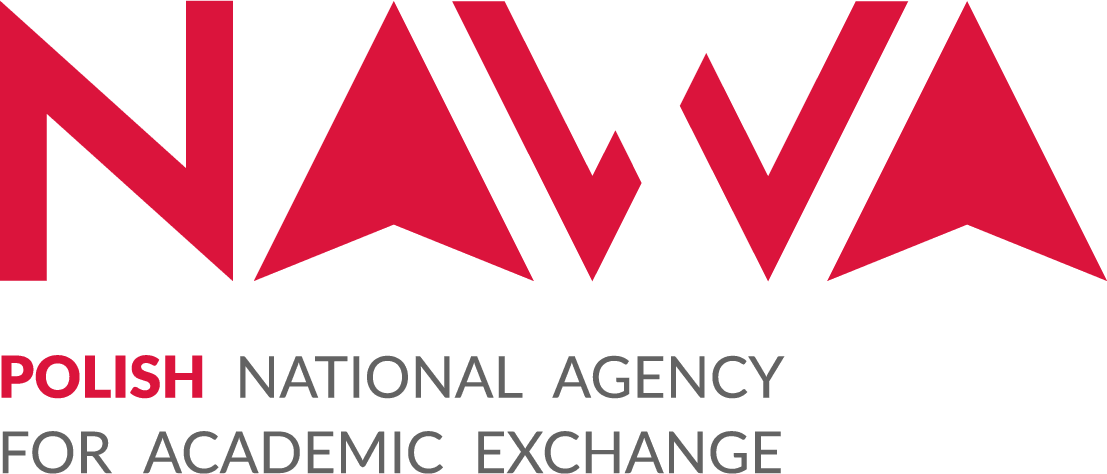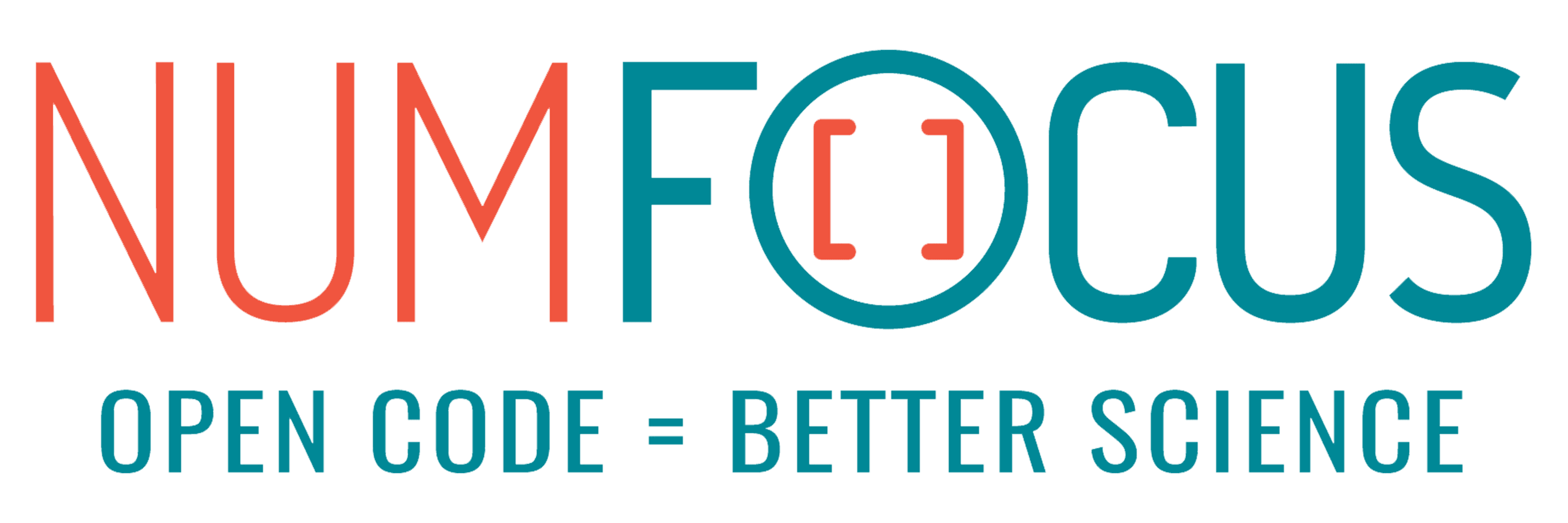# Geometric Algebra at compile-time with SymbolicGA.jl

07/27/2023, 7:00 PM7:30 PM UTC
32-144

#### Abstract:

Geometric Algebra is a high-level mathematical framework which expresses a variety of geometric computations with an intuitive language. While its rich structure unlocks deeper insight and an elegant simplicity, it often comes at a cost to numerical implementations. After giving an overview of geometric algebra and its applications, a Julia implementation is presented which uses metaprogramming to shift the work to compile-time, enabling a fast and expressive approach to computational geometry.

#### Description:

Geometric Algebra is a high-level mathematical framework which expresses a large range of geometric computations in a simple and intuitive language. From a single set of rules and axioms, this framework allows you to create diverse and geometrically meaningful spaces which best suit your needs.

Complex numbers and quaternions may be identified as elements in such spaces which describe rotations in two and three dimensions. These spaces may express Euclidean transformations, such as reflections, rotations and translations; others express intersections of flat geometry such as lines and planes, and may include rounded geometry such as circles and spheres in slightly more complex spaces - all in a dimension-agnostic manner.

The price to pay for this unifying, high-level framework is extra mathematical structure that is generally not a zero-cost abstraction. However, by shifting the application of this structure to compile-time, it is possible to combine the expressive power of geometric algebra with highly performant code.

In this talk, pragmatic motivations for considering geometric algebra are provided, with a quick introduction to its formalism. Then, the open-source SymbolicGA.jl package is presented as a compile-time implementation of geometric algebra. It will be shown that the language of geometric algebra can be used to describe many geometric operations, all with a low symbolic complexity and in a performant manner.